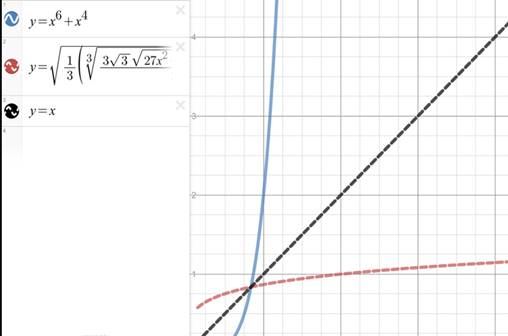# The expression for g − 1 ( x )### Single Variable Calculus: Concepts...

4th Edition
James Stewart
Publisher: Cengage Learning
ISBN: 9781337687805### Single Variable Calculus: Concepts...

4th Edition
James Stewart
Publisher: Cengage Learning
ISBN: 9781337687805

#### Solutions

Chapter 1.6, Problem 58E

(a)

To determine

## To find : The expression for g−1(x)

Expert Solution

### Explanation of Solution

Given: The given function is g(x)=x6+x40 .

Consider the function.

g(x)=x6+x40

To get the inverse replace the x with y and vice-versa in the given function.

x=y6+y4

With the help of CAS the solution obtained is,

y=±13(3327x24x+27x2323+233327x24x+27x23)13

Also,

y=±((1i3)3327x24x+27x23623+(1+i3)3×22/33327x24x+27x23)13

And,

y=±((1+i3)3327x24x+27x23623+(1i3)3×22/33327x24x+27x23)13

The four different solutions are not valid because they contain i that makes them non-rea; solutions

Therefore, the inverse function is g1(x)=±13(3327x24x+27x2323+233327x24x+27x23)13 .

(b)

To determine

Expert Solution

### Explanation of Solution

Given: The given function is g(x)=x6+x40 .

The graph of y=g(x),y=x and y=g1(x) is shown below.Figure (1)

It is visible from the figure, that the two graphs are symmetric about the line y=x .

### Have a homework question?

Subscribe to bartleby learn! Ask subject matter experts 30 homework questions each month. Plus, you’ll have access to millions of step-by-step textbook answers!